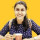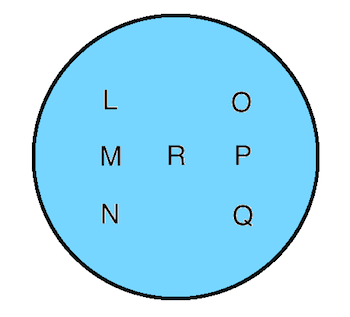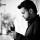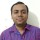1. Home >
2. Apps >
3. Groups >

# Grand Quiz Question #10

Question asked by Ankita Katdare in #Brainy Puzzles on Nov 10, 2011Ankita Katdare · Nov 10, 2011
Rank A1 - PRO
The 10th Question of the CE Grand Quiz Contest is as follows -

[Worth 5 CE Coins]Use any seven numbers from 0 to 9 to represent the letters in the figure above, such that

Value of : M * R * P = L * M * N = O * P * Q

(* represents multiplication)

Arrange the numbers in the format of the letters in the figure and write which letter represents which number.

--------------------------------------------------------------
The fastest to answer correctly wins!

Posted in: #Brainy PuzzlesAnoop Mathew · Nov 10, 2011
Rank A3 - PRO
L = 2
M = 3
N = 4
R= 8
O=6
P=1
Q=4Sahithi Pallavi · Nov 10, 2011
Rank A2 - PRO
3 1
4 2 9
6 8

and the multiplication is 72Varsha0802 · Nov 10, 2011
Rank C2 - EXPERT
L:2
m:3
n:20
r:4
o:12
p:10
q:1Anoop Mathew · Nov 10, 2011
Rank A3 - PRO
Ooops!!! I missed a number.Varsha0802 · Nov 10, 2011
Rank C2 - EXPERT
And the multiplication is 120Sahithi Pallavi · Nov 10, 2011
Rank A2 - PRO
oh no, something wrong with the editor.

L=3
M=4
N=6
R=2
O=1
P=9
Q=8

M * R * P = L * M * N = O * P * Q = 72.eternalthinker · Nov 10, 2011
Rank C2 - EXPERT
l=3 o=1
m=4 r=2 p=9
n =6 q=8Anil Jain · Nov 10, 2011
Rank A2 - PRO
L=3, m=4, n=6, r=2, o=1, p=9, q=8

3 1
4 2 9
6 8

-cbSmriti Jha · Nov 10, 2011
Rank C3 - EXPERT
L=3
m=4
n=6
o=8
p=9
q=1
r=2Mikk Sharma · Nov 10, 2011
Rank E2 - BEGINNER
i will go with anoopthefriendHarshad Italiya · Nov 10, 2011
Rank A1 - PRO